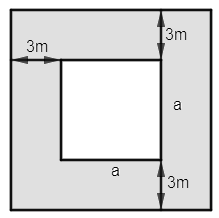QuestionAnswers

# A square park has a 3 meter wide road inside it running along its sides. The area occupied by the road is 1764 sq meters. What is the perimeter along the outer edge of the road?Hint: To find the area of the road, find the area of the whole square park including the road by taking the square of the side length along the outer edge of the road as the area of the square is given by the square of its side. From the whole area subtract the area of the square inside the road to get the area of the road and equate it with 1764 sq meters to get the side length of the inner square. Remember, the side length of the inner square is 6m less than the outer square, as 3m of road on each end is there.

Complete step by step solution:
To start with the solution we let the side length of the inner square be a meter.
Now we will draw the diagram of the situation given in the question.Now let us move to the area of the area of the road, for which side length is 6m more than that of the inner square, as 3m wide from each of the inner square. So, the side length of the outer square is (a+6) m. We also need to subtract the area of the inner square from the area of the outer square to get the area of the road. We know that the area of the square is equal to the square of its side length.
$\text{Area of the road}=\text{Area of the outer square}-\text{Area of the inner square}\text{. }$
$\Rightarrow \text{Area of the road}={{\left( a+6 \right)}^{2}}-{{a}^{2}}\text{. }$
Also, it is given that the area of the road is 1764 sq meters.
$1764={{\left( a+6 \right)}^{2}}-{{a}^{2}}\text{. }$
Now we will use the formula ${{a}^{2}}-{{b}^{2}}=\left( a+b \right)\left( a-b \right)$ . On doing so, we get
$1764=\left( a+6-a \right)\left( a+6+a \right)\text{ }$
$\Rightarrow 1764=6\left( 2a+6 \right)\text{ }$
$\Rightarrow 294=2a+6$
$\Rightarrow 2a=288$
$\Rightarrow a=144meters$
Therefore, the side length of the outer square is a+6=144+6=150 meters.
Now we know that the perimeter of a square is 4 times its side length. So, the perimeter along the outer edges is $4\times 150=600m$ .
Therefore, the answer to the above question is 600m.

Note: Remember that 1764 is not the area of the whole outer square, but it is only the area of the road, i.e., the shaded region in the figure. Also, we could have solved $1764={{\left( a+6 \right)}^{2}}-{{a}^{2}}\text{. }$ by using the formula of ${{\left( a+b \right)}^{2}}$ as well, but you need to use a formula in either case, whether you use the whole square or (a+b)(a-b), the result would be the same.

View Notes
How to Find Square Root of a NumberArea of a Sector of a Circle FormulaArea of Square FormulaArea of a Rhombus FormulaSquare Root of 576Square Root of 144Square Root of 8Surface Area of a Prism FormulaSquare Root TricksSquare Root of 289CBSE Class 10 Hindi A Question Paper 2020Hindi A Class 10 CBSE Question Paper 2009Hindi A Class 10 CBSE Question Paper 2015Hindi A Class 10 CBSE Question Paper 2016Hindi A Class 10 CBSE Question Paper 2012Hindi A Class 10 CBSE Question Paper 2010Hindi A Class 10 CBSE Question Paper 2007Hindi A Class 10 CBSE Question Paper 2013Hindi A Class 10 CBSE Question Paper 2008Hindi A Class 10 CBSE Question Paper 2014RS Aggarwal Class 8 Mathematics Solutions for Chapter-18 Area of a Trapezium and a PolygonNCERT Solutions for Class 12 Accountancy Chapter 3 - Reconstitution of a Partnership Firm: Admission of a PartnerRS Aggarwal Solutions Class 8 Chapter-18 Area of a Trapezium and a Polygon (Ex 18B) Exercise 18.2RS Aggarwal Solutions Class 10 Chapter 3 - Linear Equations in two variables (Ex 3J) Exercise 3.10 - Free PDFRD Sharma Class 10 Solutions Chapter 3 - Exercise 3.10RD Sharma Class 8 Solutions Chapter 3 - Square and Square Roots (Ex 3.7) Exercise 3.7RD Sharma Class 8 Solutions Chapter 3 - Square and Square Roots (Ex 3.5) Exercise 3.5RS Aggarwal Solutions Class 8 Chapter-18 Area of a Trapezium and a Polygon (Ex 18A) Exercise 18.1RS Aggarwal Solutions Class 10 Chapter 3 - Linear Equations in two variables (Ex 3C) Exercise 3.3RS Aggarwal Solutions Class 8 Chapter-18 Area of a Trapezium and a Polygon (Ex 18C) Exercise 18.3